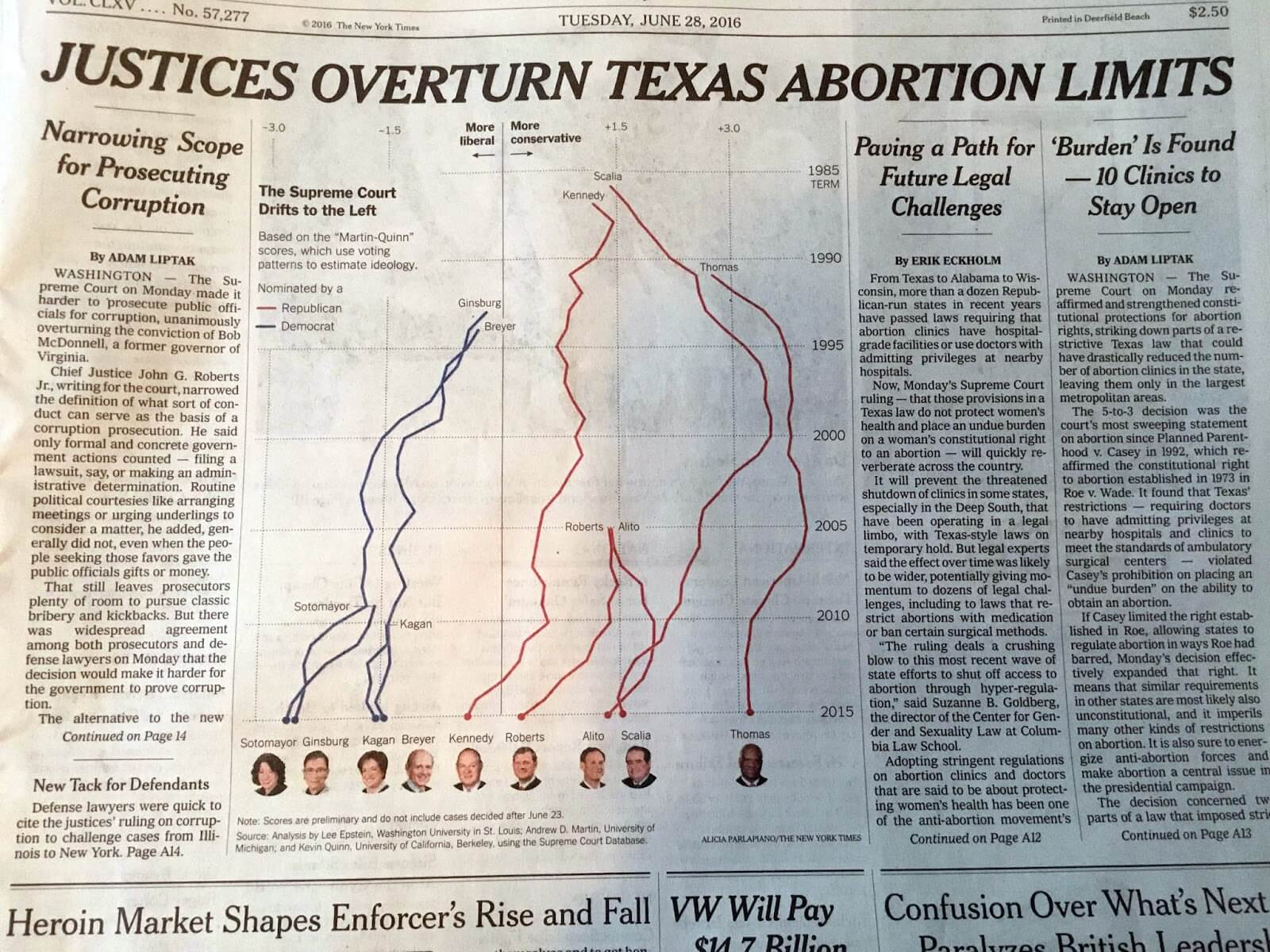Want to share your content on R-bloggers? click here if you have a blog, or here if you don't.

Once again, @albertocairo notices an interesting chart and spurs pondering in the visualization community with his post covering an unusual “vertical time series” chart produced for the print version of the NYTimes:I’m actually less concerned about the vertical time series chart component here since I agree with TAVE* Cairo that folks are smart enough to grok it and that it will be a standard convention soon enough given the prevalence of our collective tiny, glowing rectangles. The Times folks plotted Martin-Quinn (M-Q) scores for the U.S. Supreme Court justices which are estimates of how liberal or conservative a justice was in a particular term. Since they are estimates they aren’t exact and while it’s fine to plot the mean value (as suggested by the M-Q folks), if we’re going to accept the intelligence of the reader to figure out the nouveau time series layout, perhaps we can also show them some of the uncertainty behind these estimates.

What I’ve done below is take the data provided by the M-Q folks and make what I’ll call a vertical time series river plot using the mean, median and one standard deviation. This shows the possible range of real values the estimates can take and provides a less-precise but more forthright view of the values (in my opinion). You can see right away that they estimates are not so precise, but there is still an overall trend for the justices to become more liberal in modern times.The ggplot2 code is a bit intricate, which is one reason I’m posting it. You need to reorient your labeling mind due to the need to use `coord_flip()`. I also added an arrow on the Y-axis to show how time flows. I think the vis community will need to help standardize on some good practices for how to deal with these vertical time series charts to help orient readers more quickly. In a more dynamic visualization, either using something like D3 or even just stop-motion animation, the flow could actually draw in the direction time flows, which would definitely make it easier immediately orient the reader.

However, the main point here is to not be afraid to show uncertainty. In fact, the more we all work at it, the better we’ll all be able to come up with effective ways to show it.

* == “The Awesome Visualization Expert” since he winced at my use of “Dr. Cairo” 🙂

``````library(dplyr)
library(hrbrmisc) # devtools::install_github("hrbrmstr/hrbrmisc")
library(grid)
library(scales)

URL <- "http://mqscores.berkeley.edu/media/2014/justices.csv"
fil <- basename(URL)

justices %>%
filter(term>=1980,
justiceName %in% c("Thomas", "Scalia", "Alito", "Roberts", "Kennedy",
"Breyer", "Kagan", "Ginsburg", "Sotomayor")) %>%
mutate(col=ifelse(justiceName %in% c("Breyer", "Kagan", "Ginsburg", "Sotomayor"),
"Democrat", "Republican")) -> recent

just_labs <- data_frame(
label=c("Thomas", "Scalia", "Alito", "Roberts", "Kennedy", "Breyer", "Kagan", "Ginsburg", "Sotomayor"),
x=c(  1990.5,   1985.5,  2004.5,    2004.5,    1986.5,      1994,   2010,     1992.5,      2008.5),
y=c(     2.9,      1.4,    1.35,       1.7,       1.0,      -0.1,   -0.9,       -0.1,          -2)
)

gg <- ggplot(recent)
gg <- gg + geom_hline(yintercept=0, alpha=0.5)
gg <- gg + geom_label(data=data.frame(x=c(0.1, -0.1),
label=c("More →\nconservative", "← More\nliberal"),
hjust=c(0, 1)), aes(y=x, x=1982, hjust=hjust, label=label),
family="Arial Narrow", fontface="bold", size=4, label.size=0, vjust=1)
gg <- gg + geom_ribbon(aes(ymin=post_mn-post_sd, ymax=post_mn+post_sd, x=term,
group=justice, fill=col, color=col), size=0.1, alpha=0.3)
gg <- gg + geom_line(aes(x=term, y=post_med, color=col, group=justice), size=0.1)
gg <- gg + geom_text(data=just_labs, aes(x=x, y=y, label=label),
family="Arial Narrow", size=2.5)
gg <- gg + scale_x_reverse(expand=c(0,0), limits=c(2014, 1982),
breaks=c(2014, seq(2010, 1990, -10), 1985, 1982),
labels=c(2014, seq(2010, 1990, -10), "1985\nTERM\n↓", ""))
gg <- gg + scale_y_continuous(expand=c(0,0), labels=c(-2, "0\nM-Q Score", 2, 4))
gg <- gg + scale_color_manual(name=NULL, values=c(Democrat="#2166ac", Republican="#b2182b"), guide=FALSE)
gg <- gg + scale_fill_manual(name="Nominated by a", values=c(Democrat="#2166ac", Republican="#b2182b"))
gg <- gg + coord_flip()
gg <- gg + labs(x=NULL, y=NULL,
title="Martin-Quinn scores for selected justices, 1985-2014",
subtitle="Ribbon band derived from mean plus one standard deviation. Inner line is the M-Q median.",
caption="Data source: http://mqscores.berkeley.edu/measures.php")
gg <- gg + theme_hrbrmstr_an(grid="XY")
gg <- gg + theme(plot.subtitle=element_text(margin=margin(b=15)))
gg <- gg + theme(legend.title=element_text(face="bold"))
gg <- gg + theme(legend.position=c(0.05, 0.6))
gg <- gg + theme(plot.margin=margin(20,20,20,20))
gg``````

Yes, I manually positioned the names of the justices, hence the weird spacing for those lines. Also, after publishing this post, I tweaked the line-height of the “More Liberal”/”More Conservative” top labels a bit and would definitely suggest doing that to anyone attempting to reproduce this code (the setting I used was `0.9`).